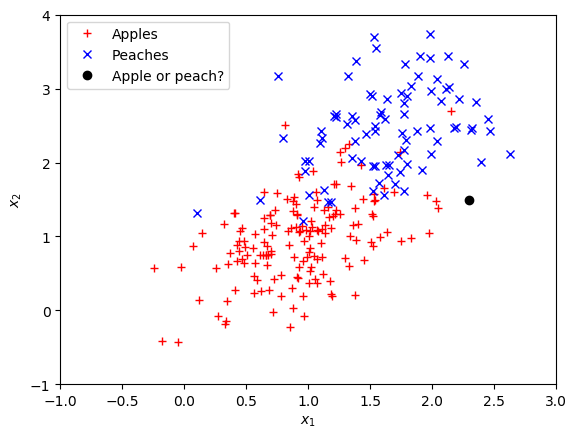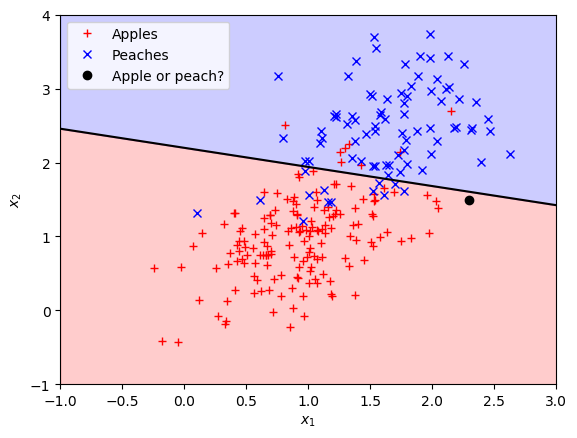# Generative Classification¶

### Preliminaries¶

• Goal

• Introduction to linear generative classification with multinomial-Gaussian generative model
• Materials

• Mandatory
• These lecture notes
• Optional
• Bishop pp. 196-202

### Example Problem: an apple or a peach?¶

You're given numerical values for the skin features roughness and color for 200 pieces of fruit, where for each piece of fruit you also know if it is an apple or a peach. Now you receive the roughness and color values for a new piece of fruit but you don't get its class label (apple or peach). What is the probability that the new piece is an apple?

In :
using Distributions, PyPlot
N = 250; p_apple = 0.7; Σ = [0.2 0.1; 0.1 0.3]
p_given_apple = MvNormal([1.0, 1.0], Σ) # p(X|y=apple)
p_given_peach = MvNormal([1.7, 2.5], Σ) # p(X|y=peach)
X = Matrix{Float64}(2,N); y = Vector{Bool}(N) # true corresponds to apple
for n=1:N
y[n] = (rand() < p_apple) # Apple or peach?
X[:,n] = y[n] ? rand(p_given_apple) : rand(p_given_peach) # Sample features
end
X_apples = X[:,find(y)]'; X_peaches = X[:,find(.!y)]' # Sort features on class
x_test = [2.3; 1.5] # Features of 'new' data point

function plot_fruit_dataset()
# Plot the data set and x_test
plot(X_apples[:,1], X_apples[:,2], "r+")   # apples
plot(X_peaches[:,1], X_peaches[:,2], "bx") # peaches
plot(x_test, x_test, "ko")           # 'new' unlabelled data point
legend(["Apples"; "Peaches"; "Apple or peach?"], loc=2)
xlabel(L"x_1"); ylabel(L"x_2"); xlim([-1,3]); ylim([-1,4])
end
plot_fruit_dataset();### Generative Classification Problem Statement¶

• Given is a data set $D = \{(x_1,y_1),\dotsc,(x_N,y_N)\}$
• inputs $x_n \in \mathbb{R}^D$ are called features.
• outputs $y_n \in \mathcal{C}_k$, with $k=1,\ldots,K$; The discrete targets $\mathcal{C}_k$ are called classes.
• We will again use the 1-of-$K$ notation for the discrete classes. Define the binary class selection variable $$y_{nk} = \begin{cases} 1 & \text{if } \, y_n \in \mathcal{C}_k\\ 0 & \text{otherwise} \end{cases}$$
• (Hence, the notations $y_{nk}=1$ and $y_n \in \mathcal{C}_k$ mean the same thing.)
• The plan for generative classification: build a model for the joint pdf $p(x,y)= p(x|y)p(y)$ and use Bayes to infer the posterior class probabilities
$$p(y|x) = \frac{p(x|y) p(y)}{\sum_{y} p(x|y) p(y)} \propto p(x|y)\,p(y)$$

### 1 - Model specification¶

##### Likelihood¶
• Assume Gaussian class-conditional distributions with constant covariance matrix across the classes, $$p(x_n|\mathcal{C}_{k}) = \mathcal{N}(x_n|\mu_k,\Sigma)$$ with notational shorthand: $\mathcal{C}_{k} \triangleq (y_n \in \mathcal{C}_{k})$.
##### Prior¶
• We use a categorical distribution for the class labels $y_{nk}$: $$p(\mathcal{C}_{k}) = \pi_k$$

• This leads to $$p(x_n,\mathcal{C}_{k}) = \pi_k \cdot \mathcal{N}(x_n|\mu_k,\Sigma)$$

• The log-likelihood for the full data set is then \begin{align*} \log\, &p(D|\theta) \stackrel{\text{IID}}{=} \sum_n \log p(x_n,\mathcal{C}_{1},\ldots,\mathcal{C}_{K} \,|\,\theta) \\ &= \sum_n \log \prod_k p(x_n,\mathcal{C}_{k}|\theta)^{y_{nk}} \;\;\text{(use 1-of-K coding)} \\ &= \sum_{n,k} y_{nk} \log p(x_n,\mathcal{C}_{k}|\theta) \\ &= \sum_{n,k} y_{nk} \log\mathcal{N}(x_n|\mu_k,\Sigma) + \sum_{n,k} y_{nk} \log \pi_k \\ &= \sum_{n,k} y_{nk} \underbrace{ \log\mathcal{N}(x_n|\mu_k,\Sigma) }_{ \text{see Gaussian est.} } + \underbrace{ \sum_k m_k \log \pi_k }_{ \text{see multinomial est.} } \end{align*} where we used $m_k \triangleq \sum_n y_{nk}$.
• As usual, the rest (inference for parameters and model prediction) through straight probability theory.

### 2 - Parameter Inference for Classification¶

• We'll do ML estimation for $\theta = \{ \pi_k, \mu_k, \Sigma \}$ from data $D$
• Recall (from the previous slide) the log-likelihood (LLH)
$$\log\, p(D|\theta) = \sum_{n,k} y_{nk} \underbrace{ \log\mathcal{N}(x_n|\mu_k,\Sigma) }_{ \text{Gaussian} } + \underbrace{ \sum_k m_k \log \pi_k }_{ \text{multinomial} }$$
• Maximization of the LLH breaks down into
• Gaussian density estimation for parameters $\mu_k, \Sigma$, since the first term contains exactly the LLH for MVG density estimation (see lesson on Density Est., Eq.1)
• Multinomial density estimation for class priors $\pi_k$, since the second term holds exactly the LLH for multinomial density estimation (see lesson on Density Estimation, Eq.2).
• The ML for multinomial class prior (we've done this before!) \begin{align*} \hat \pi_k = m_k/N \end{align*}
• Now group the data into separate classes and do MVG ML estimation for class-conditional parameters (we've done this before as well): \begin{align*} \hat \mu_k &= \frac{ \sum_n y_{nk} x_n} { \sum_n y_{nk} } = \frac{1}{m_k} \sum_n y_{nk} x_n \\ \hat \Sigma &= \frac{1}{N} \sum_{n,k} y_{nk} (x_n-\hat \mu_k)(x_n-\hat \mu_k)^T \\ &= \sum_k \hat \pi_k \cdot \underbrace{ \left( \frac{1}{m_k} \sum_{n} y_{nk} (x_n-\hat \mu_k)(x_n-\hat \mu_k)^T \right) }_{ \text{class-cond. variance} } \\ &= \sum_k \hat \pi_k \cdot \hat \Sigma_k \end{align*} where $\hat \pi_k$, $\hat{\mu}_k$ and $\hat{\Sigma}_k$ are the sample proportion, sample mean and sample variance for the $k$th class, respectively.
• Note that the binary class selection variable $y_{nk}$ groups data from the same class.

### 3 - Application: Class prediction for new Data¶

• Let's apply the trained model: given a 'new' input $x_\bullet$, use Bayes rule to get posterior class probability \begin{align*} p(\mathcal{C}_k|x_\bullet,\theta ) &\propto p(\mathcal{C}_k) \,p(x_\bullet|\mathcal{C}_k) \\ &\propto \pi_k \exp \left\{ { - {\frac{1}{2}}(x_\bullet - \mu_k )^T \Sigma^{ - 1} (x_\bullet - \mu_k )} \right\}\\ &\propto \exp \left\{ {\mu_k^T \Sigma^{ - 1} x_\bullet - {\frac{1}{2}}\mu_k^T \Sigma^{ - 1} \mu_k + \log \pi_k } \right\} \\ &= \exp\{\beta_k^T x + \gamma_k\} \end{align*} where \begin{align*} \beta_k &= \Sigma^{-1} \mu_k \\ \gamma_k &= - \frac{1}{2} \mu_k^T \Sigma^{-1} \mu_k + \log \pi_k \,. \end{align*}
• The class posterior function $$\phi(a_k) \triangleq \frac{\exp(a_k)}{\sum_{k^\prime}\exp(a_{k^\prime})}$$ is called a softmax function. Note that the softmax function is per definition properly normalized in the sense that $\sum_k \phi(a_k) = 1$.

### Discrimination Boundaries¶

• The class log-posterior $\log p(\mathcal{C}_k|x) \propto \beta_k^T x + \gamma_k$ is a linear function of the input features.
• Thus, the contours of equal probability (discriminant functions) are lines (hyperplanes) in feature space" $$\log \frac{{p(\mathcal{C}_k|x,\theta )}}{{p(\mathcal{C}_j|x,\theta )}} = \beta_{kj}^T x + \gamma_{kj} = 0$$ where we defined $\beta_{kj} \triangleq \beta_k - \beta_j$ and similarly for $\gamma_{kj}$.
• (homework). What happens if we had not assumed class-independent variances $\Sigma_k=\Sigma$? Are the discrimination functions still linear? quadratic?
• How to classify a new input $x_\bullet$? The Bayesian answer is a posterior distribution $p(\mathcal{C}_k|x_\bullet)$. If you must choose, then the class with maximum posterior class probability \begin{align*} k^* &= \arg\max_k p(\mathcal{C}_k|x_\bullet) \\ &= \arg\max_k \left( \beta _k^T x_\bullet + \gamma_k \right) \end{align*} is an appealing decision.

#### CODE EXAMPLE¶

We'll apply the above results to solve the "apple or peach" example problem.

In :
# Make sure you run the data-generating code cell first

# Multinomial (in this case binomial) density estimation
p_apple_est = sum(y.==true) / length(y)
π_hat = [p_apple_est; 1-p_apple_est]

# Estimate class-conditional multivariate Gaussian densities
d1 = fit_mle(FullNormal, X_apples')  # MLE density estimation d1 = N(μ₁, Σ₁)
d2 = fit_mle(FullNormal, X_peaches') # MLE density estimation d2 = N(μ₂, Σ₂)
Σ = π_hat*cov(d1) + π_hat*cov(d2) # Combine Σ₁ and Σ₂ into Σ
conditionals = [MvNormal(mean(d1), Σ); MvNormal(mean(d2), Σ)] # p(x|C)

# Calculate posterior class probability of x∙ (prediction)
function predict_class(k, X) # calculate p(Ck|X)
norm = π_hat*pdf(conditionals,X) + π_hat*pdf(conditionals,X)
return π_hat[k]*pdf(conditionals[k], X) ./ norm
end
println("p(apple|x=x∙) = $(predict_class(1,x_test))") # Discrimination boundary of the posterior (p(apple|x;D) = p(peach|x;D) = 0.5) β(k) = inv(Σ)*mean(conditionals[k]) γ(k) = -0.5 * mean(conditionals[k])' * inv(Σ) * mean(conditionals[k]) + log(π_hat[k]) function discriminant_x2(x1) # Solve discriminant equation for x2 β12 = β(1) - β(2) γ12 = (γ(1) - γ(2))[1,1] return -1*(β12*x1 + γ12) ./ β12 end plot_fruit_dataset() # Plot dataset x1 = linspace(-1,3,10) plot(x1, discriminant_x2(x1), "k-") # Plot discrimination boundary fill_between(x1, -1, discriminant_x2(x1), color="r", alpha=0.2) fill_between(x1, discriminant_x2(x1), 4, color="b", alpha=0.2);p(apple|x=x∙) = 0.6092722305430905  ### Recap Generative Classification¶ • Model speccification:$p(x,\mathcal{C}_k|\,\theta) = \pi_k \cdot \mathcal{N}(x|\mu_k,\Sigma)$• If the class-conditional distributions are Gaussian with equal covariance matrices across classes ($\Sigma_k = \Sigma$), then the discriminant functions are hyperplanes in feature space. • ML estimation for$\{\pi_k,\mu_k,\Sigma\}$breaks down to simple density estimation for Gaussian and multinomial. • Posterior class probability is a softmax function $$p(\mathcal{C}_k|x,\theta ) \propto \exp\{\beta_k^T x + \gamma_k\}$$ where$\beta _k= \Sigma^{-1} \mu_k$and$\gamma_k=- \frac{1}{2} \mu_k^T \Sigma^{-1} \mu_k + \log \pi_k\$.

The cell below loads the style file

In :
open("../../styles/aipstyle.html") do f